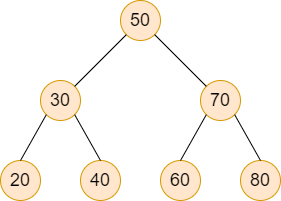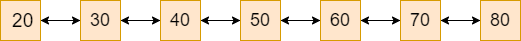# BST to sorted DLL

Posted: 17 Mar, 2021
Difficulty: Moderate

## PROBLEM STATEMENT

#### For Example:#### Consider the above BST, it will be converted into the below sorted DLL.#### Here, 20 is the head node and 80 is the tail node.

##### Input Format:
``````The first line contains a single integer ‘T’ representing the number of test cases.

The first line of each test case contains elements of the tree in the level order form. The line consists of values of nodes separated by a single space. In case a node is null, we take -1 in its place.

For example, the input for the tree depicted in the below image would be :
````````````1
2 3
4 -1 5 6
-1 7 -1 -1 -1 -1
-1 -1
``````

#### Explanation :

``````Level 1 :
The root node of the tree is 1

Level 2 :
Left child of 1 = 2
Right child of 1 = 3

Level 3 :
Left child of 2 = 4
Right child of 2 = null (-1)
Left child of 3 = 5
Right child of 3 = 6

Level 4 :
Left child of 4 = null (-1)
Right child of 4 = 7
Left child of 5 = null (-1)
Right child of 5 = null (-1)
Left child of 6 = null (-1)
Right child of 6 = null (-1)

Level 5 :
Left child of 7 = null (-1)
Right child of 7 = null (-1)

The first not-null node(of the previous level) is treated as the parent of the first two nodes of the current level. The second not-null node (of the previous level) is treated as the parent node for the next two nodes of the current level and so on.
The input ends when all nodes at the last level are null(-1).
``````
##### Note :
``````The above format was just to provide clarity on how the input is formed for a given tree.
The sequence will be put together in a single line separated by a single space according to the Binary Search tree. Hence, for the above-depicted tree, the input will be given as:

1 2 3 4 -1 5 6 -1 7 -1 -1 -1 -1 -1 -1
``````
##### Output Format:
``````For each test case, print the elements of the sorted doubly linked list in the space-separated form.

Output for every test case will be printed in a separate line.
``````
##### Note:
``````You don’t need to print anything; It has already been taken care of. Just implement the given function.
``````
``````Try to do the problem in order O(N) time complexity or less for getting the solution accepted.
``````
##### Constraints:
``````1 <= T <= 10
0 <= N <= 10^4
-10^5 <= DATA <= 10^5

Time Limit: 1sec
``````Approach 1

The basic idea of this approach is to use the Inorder tree traversal technique to traverse from the leftmost node to the rightmost node and during the traversal maintain the DLL by converting subtree into the small doubly linked list.

We will use an additional function that performs inorder traversal on the tree

``TreeNode<int>* inorderTraversal(TreeNode<int>* root, TreeNode<int>* &prev)``

Where ‘ROOT’ is the root node of BST, and ‘PREV’ is the previously traversed node.

The steps are as follows:

1. In the ‘IN_ORDER_TRAVERSAL’ function
• Check if ‘ROOT’ is ‘NULL’ then returns the root.
• Create a pointer variable ‘HEAD’ of type TREE_NODE<int> that will point to the head of DLL.
• As we must have to set this 'HEAD' to the leftmost node in the tree and so, we will make a recursive call to ROOT→LEFT until it reaches the leftmost child.
• After that, we check if 'PREV' is ‘NULL’ which means we haven’t picked any node yet, then we set our ‘HEAD’ to the ‘ROOT’.
• Else, all we have to do is to set 'ROOT' in the right of ‘PREV’ and ‘PREV’ in the left of 'ROOT'.
• Now, we have to move towards other nodes in the tree and so, we set ‘PREV' to ‘ROOT'.
• Make a recursive call for the right subtree.